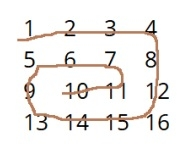# Print matrix in antispiral form

CServer Side ProgrammingProgramming

Given a 2d array of n*n and the task is to find the antispiral arrangement of the given matrix

Input : arr={1,2,3,4,
5,6,7,8,
9,10,11,12
13,14,15,16}
Output: 10 11 7 6 5 9 13 14 15 16 12 8 4 3 2 1For this, stack can be used where the transpose of a matrix can be pushed inside stack and poped reversely

## Algorithm

START
STEP 1 -> declare stack vector element as stk and variables as int r=4, c=4, i, j, rs=0 and cs=0
Step 2 -> store matrix elements in 2-3 array
Step 3 -> Loop For i=0 and o<4 and i++
Loop For j=0 and j<4 and j++
Print arr[i][j]
End
Print \n
End
Step 4 -> Loop While rs<c and cs<r
Loop For i=rs and i<c and i++
Push arr[rs][i]
End
cs++
Loop For i=cs and i<r-1 and ++i
Push arr[r-1][i]
End
c—
IF(cs<r)
Loop For i=r-1 and i>=rs and –i
Push arr[r-1][i]
End
r- -
End
IF(rs<c)
Loop For i=c-1 and i>=cs and i- -
Push arr[i][rs]
End
Rs++
End
End
Step 5 -> Loop While !stk.empty()
Print stk.top()
Call pop()
End
STOP

## Example

#include<iostream>
#include <bits/stdc++.h>
using namespace std;
int main(){
stack <int> stk;
int R=4,C=4,i,j,RS=0,CS=0;
int mat[R][C] = { {1,2,3, 4}, {5,6,7,8},{9,10,11,12},{13,14,15,16}};
for(i=0;i<4;i++){
for(j=0;j<4;j++)
cout<<mat[i][j]<<" ";
cout<<"\n";
}
while(RS<C&&CS<R) {
for(i=RS;i<C;i++)
stk.push(mat[RS][i]);
CS++;
for(i=CS;i<R-1;++i)
stk.push(mat[i][C-1]);
C--;
if(CS<R){
for(i=R-1;i>=RS;--i)
stk.push(mat[R-1][i]);
R--;
}
if(RS<C){
for(i=C-1;i>=CS;i--)
stk.push(mat[i][RS]);
RS++;
}
}
while(!stk.empty()){
cout<<stk.top()<<" ";
stk.pop();
}

## Output

if we run the above program then it will generate the following output

1 2 3 4
5 6 7 8
9 10 11 12
13 14 15 16
10 11 7 6 5 9 13 14 15 16 12 8 4 3 2 1